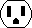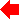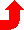Chapter 10: The JELIB and DELIB File Format10-3: Body 10-3-2: Node InstancesInside of a cell definition, node instances are declared with the "N" and "I" lines. "N" is for primitive nodes and "I" is for cell instances. All nodes are sorted by the node name. The syntax is:

 N | | | | | | | | [ | ]* I | | | | | | |
[ | ]* the type of the node instance. For primitive node instances, this has the form: [:]. If is omitted, the technology of the cell is assumed. For cell instances, it has the form: [:];{}. If is omitted, the library defined by this JELIB file is assumed. the name of the node instance. a text descriptor for the name (when displayed). the X coordinate of the anchor point of the node instance. the Y coordinate of the anchor point of the node instance. the difference between width of the primitive node and the standard width of this primitive the difference between height of the primitive node and the standard height of this primitive the orientation of the node (see below). flags for the node instance (see below). a text descriptor for the cell instance name (does not apply to primitives). a list of variables on the node instance (see Section 10-4-1).

The <orientation> field is any of the following letters, followed by an optional numeric part:

"X" if the node instance is X-mirrored (mirrored about Y axis).
"Y" if the node instance is Y-mirrored (mirrored about X axis).
"R" each letter rotates the node instance at 90 degrees counter-clockwise.
Num Any digits at the end are additional rotation in tenths of a degree.

The <flags> field is any of the following letters, sorted alphabetically, followed by a numeric part:

"A" if the node instance is hard-to-select.
"L" if the node instance is locked.
"V" if the node instance is visible only inside the cell.
Num Any digits at the end are the technology-specific bits.

Examples:

`Nschematic:Transistor|mos@0||2|0|||R|2|ATTR_length(D5G0.5;X-0.5;Y-1;)S2`
Places a schematic Transistor called "mos@0" at (2,0), standard size, rotated 90 degrees. The flag field "2" is numeric, and therefore is technology-specific information (in this case, it makes the transistor be pMOS). There is one attribute on the node, called "length", with the value "2" (a string). This attribute is displayed, anchored at its center ("D5"), is 1 half grid unit in size ("G0.5;"), and is offset (-0.5, -1) from the node center ("X-0.5;Y-1;").

`Ilow;1{lay}|HAPPY||14|12|Y|A|D5G4;`
Places an instance of cell "low{lay}" from the library defined in this JELIB file. The instance is named "HAPPY". It is at (14,12), mirrored in Y, and rotated 0. The "A" means that the node is hard-to-select. Its name is described by D5G4; (D5 for a centered anchor point; G4 for 4 units size).PreviousTable of Contents Next